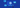# You don't need these many state variables

react
javascript
performance

24 Sep, 2022

React is reactive because of its reactive state variables and props. What if I told you, you don't need as many state variables as you require? If you've used VueJs or KnockoutJS you might know about `computed` variables. In react, most of the reactive states could be converted into computeds' without involving a state variable.

## Computed variables in React?

What is a computed variable? Computed variable is nothing but a value which is derived from or computed from a state variable. The value of this variable changes when there is a change in the state variable that it is dependent upon.

### Use of a computed variable

Computed variables can replace your extra state variables and help you reduce your re-renders. It also reduces your `useEffect` hooks that you would require to update these values.

### How to convert state variable to a computed value?

Consider this example where you have to show a error message based on the input value. You could see yourself writing two state variables. One for `value` and the other for the `error` state. The following code illustrates it.

```//bad const [value, setValue] = useState() const [error, setError] = useState() // this is not required as a state useEffect(() => { if (value === '') setError('Value cannot be empty') }, [value]) return ( <div> <input value={value} onChange={({ target }) => setValue(target.value)} /> {error && <div className="error-msg">{error}</div>} </div> ) ```

Actually you could keep the `error` as a normal variable which would be computed during each render based on the value of the `value` state.

This would be the better approach ✅

```//good const [value, setValue] = useState() // here error is a computed value const error = value === '' ? 'Value cannot be empty' : '' return ( <div> <input value={value} onChange={({ target }) => setValue(target.value)} /> {error && <div className="error-msg">{error}</div>} </div> ) ```

Thus we have eliminated a state variable and an unwanted useEffect hook. Mission accomplished. Likewise, in your daily work, identify which state variables are computed values and replace them with normal variables. Your app could become a lot faster.

⚡️⚡️⚡️

Consider subscribing to the newsletter for more tips and hacks like this.
00:00
Can you stay a bit longer?